# 简单分享下

## 1. 开篇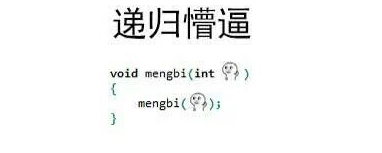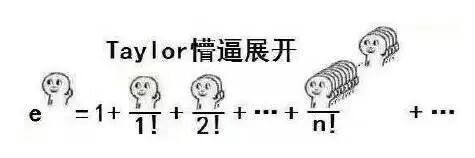## 2. 案例

2.1 反义词消消乐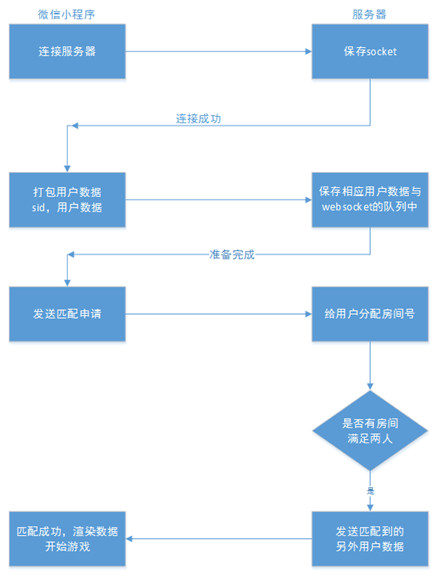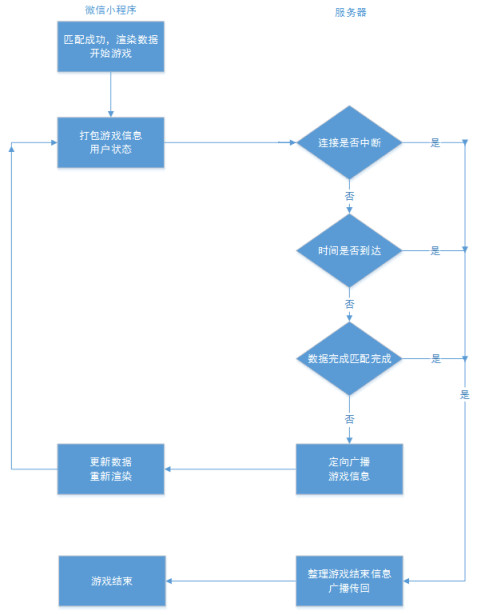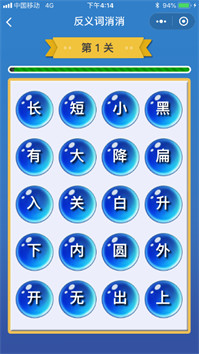2.2 困住小星星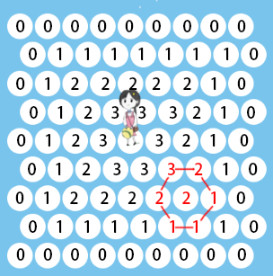``````calPath: function(location) {     //计算路径
var row = location.row
var col = location.col
if (this.data.map_location[row][col] == 1) {      //墙直接返回100
location.path = 100
return location.path
}
if (row == 0 || col == 0 || row == 8 || col == 8) {      //边界直接返回0
location.path = 0
return location.path
}
var sixDir = this.calSixDir(location)     //寻找周围的六个点

//遍历周围6个点，找出最小值
var min = 100
for (var i = 0; i < 6; i++) {
if (sixDir[i].path >= 0) {           // 存在点没有值，那需要等下次再来计算其值
var tmp = sixDir[i].path
if (min > tmp) {
min = tmp
}
}
}
if (min < 100) {
location.path = min + 1
} else {
location.path += 1
}

return location.path
},
``````2.3 fly 拖鞋、摩斯密码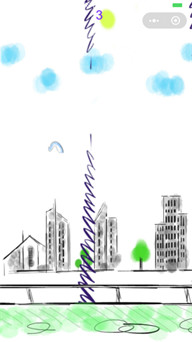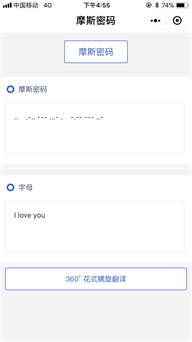## 3.聊聊

3.1 开发

3.2 收尾12 个评论